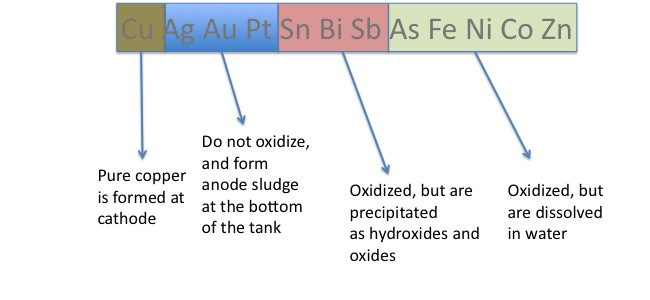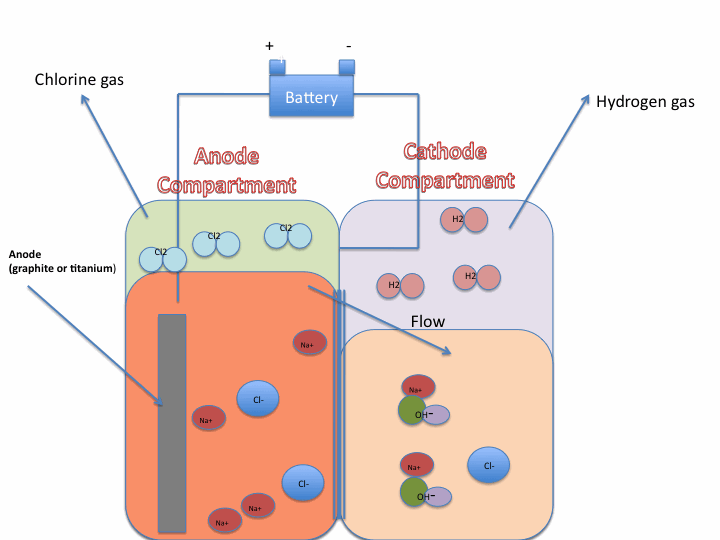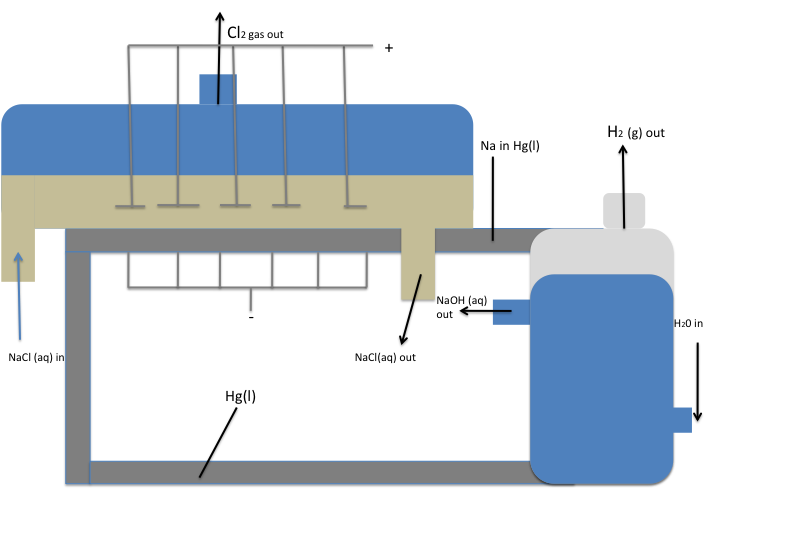# Case Study: Industrial Electrolysis

$$\newcommand{\vecs}{\overset { \rightharpoonup} {\mathbf{#1}} }$$ $$\newcommand{\vecd}{\overset{-\!-\!\rightharpoonup}{\vphantom{a}\smash {#1}}}$$$$\newcommand{\id}{\mathrm{id}}$$ $$\newcommand{\Span}{\mathrm{span}}$$ $$\newcommand{\kernel}{\mathrm{null}\,}$$ $$\newcommand{\range}{\mathrm{range}\,}$$ $$\newcommand{\RealPart}{\mathrm{Re}}$$ $$\newcommand{\ImaginaryPart}{\mathrm{Im}}$$ $$\newcommand{\Argument}{\mathrm{Arg}}$$ $$\newcommand{\norm}{\| #1 \|}$$ $$\newcommand{\inner}{\langle #1, #2 \rangle}$$ $$\newcommand{\Span}{\mathrm{span}}$$ $$\newcommand{\id}{\mathrm{id}}$$ $$\newcommand{\Span}{\mathrm{span}}$$ $$\newcommand{\kernel}{\mathrm{null}\,}$$ $$\newcommand{\range}{\mathrm{range}\,}$$ $$\newcommand{\RealPart}{\mathrm{Re}}$$ $$\newcommand{\ImaginaryPart}{\mathrm{Im}}$$ $$\newcommand{\Argument}{\mathrm{Arg}}$$ $$\newcommand{\norm}{\| #1 \|}$$ $$\newcommand{\inner}{\langle #1, #2 \rangle}$$ $$\newcommand{\Span}{\mathrm{span}}$$$$\newcommand{\AA}{\unicode[.8,0]{x212B}}$$

Electrolysis reactions are the basic foundations of today's modern industry. There are various elements, chemical compounds, and organic compounds that are only produced by electrolysis including aluminum, chlorine, and NaOH. Electrolysis is the process by which an electric current spurs an otherwise non-spontaneous reaction.

## Electrorefining: How It Works

The electrorefining process refines metals or compounds at a high purity for a low cost. The pure metal can coat an otherwise worthless object.

Let's consider the electrorefining process of copper: At the anode, there is an impure piece of copper that has other metals such as Ag, Au, Pt, Sn, Bi, Sb, As, Fe, Ni, Co, and Zn. The copper in this impure ore is oxidized to form Cu2+ at the anode, and moves through an aqueous sulfuric acid-Copper (II) sulfate solution into the cathode. When it reaches the cathode, the Cu2+ is reduced to Cu. This whole process takes place at a fairly low voltage (about .15 to .30 V), so Ag, Au, and Pt are not oxidized at the anode, as their standard oxidation electrode potentials are -.800, -1.36 and -1.20 respectively; these unoxidized impurities turn into a mixture called anode mud, a sludge at the bottom of the tank. This sludge can be recovered and used in different processes. Unlike Ag, Au and Pt, the impurities of Sb, Bi and Sn in the ore are indeed oxidized at the anode, but they are precipitated as they form hydroxides and oxides. Finally, Fe, Ni, Co and Zn are oxidized as well, but they are dissolved in water. Therefore, the only solid we are left with is the pure solid copper plate at the cathode, which has a purity level of about 99.999%. The image below gives an outline about the fate of the main components of an impure iron ore.## Electrosynthesis

Electrosynthesis is the method of producing substances through electrolysis reactions. This is useful when reaction conditions must be carefully controlled. One example of electrosynthesis is that of MnO2, Manganese dioxide. MnO2 occurs naturally in the form of the mineral pyrolusite, but this mineral is not easily used due to the nature of its size and lattice structure. However, MnO2 can be obtained a different way, through the electrolysis of MnSO4 in a sulfuric acid solution.

\begin{align} &\textrm{Oxidation: } &&\mathrm{Mn^{2+} + 2H_2O \rightarrow MnO_2 + 4H^+ + 2e^-} &&\mathrm{\hspace{12px}E^0_{MnO_2/H_2}=-1.23}\\ &\textrm{Reduction: } &&\mathrm{2e^- + 2H^+ \rightarrow H_2} &&\mathrm{{-E}^0_{H^+/H_2}= -0}\\ &\textrm{Overall: } &&\mathrm{Mn^{2+} + 2H_2O \rightarrow MnO_2 + 2H^+ +H_2} &&\mathrm{\hspace{12px}E^0_{MnO_2/H_2} -E^0_{H^+/H_2}= -1.23 - 0= -1.23} \end{align}

The commercial process for organic chemicals that is currently practiced on a scale comparable to that of inorganic chemicals and metals is the electrohydrodimerization of acrylonitrile to adiponitrile.

\begin{align} &\textrm{Anode: } &&\mathrm{H_2O \rightarrow 2H^+ + \dfrac{1}{2} O_2 + 2e^-}\\ &\textrm{Cathode: } &&\ce{2CH2=CHCN + 2H2O + 2e- \rightarrow NC(CH2)CN + 2OH-}\\ &\textrm{Overall: } &&\textrm{(acrylonitrile) }\ce{2CH2=CHCN + H2O \rightarrow \dfrac{1}{2} O2 + NC(CH2)4CN}\:\textrm{(adiponitrile)} \end{align}

The importance of adiponitrile is that it can be readily converted to other useful compounds.

## The Chlor-Alkali Process

This process is the electrolysis of sodium chloride (NaCl) at an industrial level. We will begin by discussing the equation for the chlor-alkali process, followed by discussing three different types of the process: the diaphragm cell, the mercury cell and the membrane cell.

### What is the equation for the electrolysis of NaCl?

We will begin the explanation of the chlor-alkali process by determining the reactions that occur during the electrolysis of NaCl. Because NaCl is in an aqueous solution, we also have to consider the electrolysis of water at both the anode and the cathode. Therefore, there are two possible reduction equations and two possible oxidation reactions.

Reduction:

\begin{align}
& \mathrm{Na^+_{\large{(aq)}} + 2e^- \rightarrow Na_{\large{(s)}}}
&& \mathrm{E^0_{Na^+/Na}= -2.71\: V \tag{1}}\\
& \mathrm{2 H_2O_{\large{(l)}} + 2e^- \rightarrow H_{2\large{(g)}} +2OH^-_{\large{(aq)}}}
&& \mathrm{E^0_{H_2O/H_2}= -.83\: V \tag{2}}
\end{align}

Oxidation:

\begin{align} &\mathrm{2Cl^-_{\large{(aq)}} \rightarrow Cl_2 + 2e^-} &&\mathrm{-E^0_{Cl_2/Cl^-}= -(1.36\: V) \tag{3}}\\ &\mathrm{2H_2O_{\large{(l)}} \rightarrow O_2 + 4H^+ + 4e^-} &&\mathrm{-E^0_{O_2/H_2O}= -(1.23\: V) \tag{4}} \end{align}

As we can see, due to the very much more negative electrode potential, the reduction of sodium ions is much less likely to occur than the reduction of water, so we can assume that in the electrolysis of NaCl, the reduction that occurs is reaction (2). Therefore, we should try to determine what the oxidation reaction that occurs is. Let's say we have reaction (2) as the reduction and reaction (3) as the oxidation. We would get:

\begin{align} &\textrm{Reduction: } &&\mathrm{2 H_2O_{\large{(l)}} + 2e^- \rightarrow H_{2\large{(g)}} +2OH^-_{\large{(aq)}}} &&\mathrm{\hspace{12px}E^0_{H_20/H_2}= -.83\: V \tag{2}}\\ &\textrm{Oxidation: } &&\mathrm{2Cl^-_{\large{(aq)}} \rightarrow Cl_2 + 2e^-} &&\mathrm{-E^0_{Cl_2/Cl^-}= -(1.36\: V) \tag{3}} \\ &\textrm{Overall: } &&\mathrm{2 H_2O_{\large{(l)}} + 2Cl^-_{\large{(aq)}}} &&\mathrm{\hspace{12px}E^0_{H_20/H_2} - E^0_{Cl_2/Cl^-}\tag{5}}\\ & &&\mathrm{\hspace{10px}\rightarrow H_{2\large{(g)}} +2OH^-_{\large{(aq)}} +Cl_2} &&\mathrm{\hspace{22px} = -.83 + (-1.36)= -2.19} \end{align}

On the other hand, we could also have reaction (2) with reaction (4)

\begin{align} &\textrm{Reduction: } &&\mathrm{2\,[2 H_2O_{\large{(l)}} + 2e^- \rightarrow H_{2\large{(g)}} +2OH^-_{\large{(aq)}}]} &&\mathrm{\hspace{12px}E_{H_2O/H_2O}=-.83\: V \tag{2}}\\ &\textrm{Oxidation: } &&\mathrm{2H_2O_{\large{(l)}} \rightarrow O_2 + 4H^+ + 4e^-} &&\mathrm{-E_{O_2/H_20}^0= -(1.23\: V) \tag{4}}\\ &\textrm{Overall: } &&\mathrm{2H_2O_{\large{(l)}} \rightarrow 2H_{2\large{(g)}} + O_{2\large{(g)}}} &&\mathrm{\hspace{12px}E^0_{H_2O/H_2} - E^0_{O_2/H_20}\tag{6}}\\ & && && \mathrm{\hspace{22px}= -.83\: V -(1.23\: V)= -2.06} \end{align}

At first glance it would appear as though reaction (6) would occur due to the smaller (less negative) electrode potential. However, O2 actually has a fairly large overpotential, so instead Cl2 is more likely to form, making reaction (5) the most probable outcome for the electrolysis of NaCl.

### Chlor-Alkali Process in a Diaphragm Cell

Depending on the method used, there can be several different products produced through the chlor-alkali process. The value of these products is what makes the chlor-alkali process so important. The name comes from the two main products of the process, chlorine and the alkali, sodium hydroxide (NaOH). Therefore, one of the main uses of the chlor-alkali process is the production of NaOH. As described earlier, the equation for the chlor-alkali process, that is, the electrolysis of NaCl, is as follows:

\begin{align} &\textrm{Reduction: } &&\mathrm{2 H_2O_{\large{(l)}} + 2e^- \rightarrow H_{2\large{(g)}} +2OH^-_{\large{(aq)}}} &&\mathrm{E_{H_2O/H_2O}= -.83}\\ &\textrm{Oxidation: } &&\mathrm{2Cl^-_{\large{(aq)}} \rightarrow Cl_2 + 2e^-} &&\mathrm{E^0_{Cl_2/Cl^-}= -1.36}\\ &\textrm{Overall: } &&\mathrm{2Cl^- + 2H_2O_{\large{(l)}} \rightarrow 2 OH^- + H_{2\large{(g)}} +Cl_{2\large{(g)}}} &&\mathrm{E^0_{H_2O/H_2}- E^0_{Cl/l2^-}}\\ & && &&\hspace{12px}\mathrm{= -83\:V- (-1.36\: V)= -2.19\: V} \end{align}

**The chlor-alkali process often occurs in an apparatus called a diaphragm cell, which is illustrated below.Figure 1:
As you look at the cell, note the following things:

Anode

• The sodium chloride is put into the anode compartment, in aqueous form.
• The actual physical anode is made of either graphite or titanium.
• In the anode compartment, Cl2 gas is produced as Cl- is oxidized.

Cathode

• On the cathode side, OH- (aq) and H2 gas are formed as water is reduced.
• You may wonder, why are there sodium and chloride ions of the cathode side if we put the sodium chloride into the anode compartment? To answer this question, we can consider the difference in solution levels between the anode and cathode. This causes the gradual flow of NaCl into the cathode, and prevents the backflow of NaOH into the anode. If Cl2 and NaOH come into contact, Cl2 turns into ClO-, ClO3-, Cl- ions. The water level difference prevents this contact and also encourages the flow of NaCl to the cathode side, so NaOH (aq) can form.
• Now, you may notice that the solution with NaOH in the cathode will also have aqueous NaCl mixed with it due to the flow of NaCl from the anode to the cathode. Therefore, if we want very pure NaOH for uses such as rayon manufacture, we need to somehow purify the salt out of the NaOH. In general, before purification, the solution is about 14-16% NaCl (aq) and 10-12% NaOH (aq). However, the NaOH (aq) can be concentrated and the NaCl (s) can be crystallized to form a solution with 50% NaOH (aq) and about 1% NaCl (aq).

### Chlor-Alkali Process in Mercury Cell

To even further improve the purity of NaOH, a mercury cell can be used for the location of electrolysis, opposed to a diaphragm cell.Figure 2:

Anode Side

• The anodes are placed in the aqueous NaCl solution, above the liquid mercury.
• The reduction of Cl- occurs to produce chlorine gas, Cl2 (g).

Cathode Side

• A layer of Hg (l) at the bottom of the tank serves as the cathode.
• With a mercury cathode, the reaction of H2O (l) to H2 has a fairly high over potential, so the reduction of Na+ to Na occurs instead. The Na is soluble in Hg (l) and the two combine to form the Na-Hg alloy amalgam. This amalgam can be removed and then mixed with water to cause the following reaction:
• $$\ce{2Na\:(in\: Hg) + 2H2O \rightarrow 2 Na+ + 2 OH- + H_{2\large{(g)}} + Hg_{\large{(l)}}}$$
• The Hg (l) that forms is recycled back into the liquid at the bottom of the tank that acts as a cathode.
• H2 gas is released.
• NaOH is left in a very pure, aqueous form.

**Some of the main problems with the mercury cell are as follows:

• The reaction needs a higher voltage than the diaphragm cell: 4.5 V in the mercury cell compared to 3.5 in a diaphragm cell.
• Requires quite a bit of electrical energy, as it needs 3400 kWh/ton Cl2 opposed to 2500 in a diaphragm cell.
• Potential damage to the environment due to mercury deposits. Luckily deposits were as large as 200 g mercury per ton chlorine gas, but now, they never exceed 0.25 g per ton chlorine gas.

### Membrane Cell Process

One final way to make even more pure NaOH is to use a membrane cell. It is preferred over the diaphragm cell or mercury cell method because it uses the least amount of electric energy and produces the highest quality NaOH. For instance, it can produce NaOH with a degree of chlorine ion contamination of only 50 parts per million. An ion-permeable membrane is used to separate the anode and cathode.

Anode

• Saturated brine is fed to the compartment.
• Current passed through the cell splits the sodium chloride into its constituent components, Na+ and Cl-.
• Chloride ions are oxidized to chlorine gas at the anode.
• The membrane passes Na+ ions to the cathode compartment.

Cathode

• H2O is reduced to form OH- and H2 gas.
• $$\ce{2H2O \rightarrow H2 + 2OH- }$$
• Na+ ions that had flowed over and OH- produced through the reduction of water react to form aqueous NaOH.
• H2 gas also is produced as a byproduct.True or False:

1. The main product of the chlor-alkali process is NaCl.
2. A diaphragm cell yields the purest concentration of NaOH.
3. In a diaphragm cell, the oxidation of Cl- ions occurs at the anode and the reduction of Na+ occurs at the cathode.
4. H2 gas is a byproduct of the chlor-alkali process.
5. Electrorefining for copper is effective because out of the all of the metals in an ore, only copper is oxidized at the anode at low voltages.
6. A mercury cell uses mercury liquid as the cathode.
7. The chlor-alkali process is spontaneous.
8. A mercury cell requires a higher voltage and more energy than a diaphragm cell.
9. In a membrane cell, all ions can freely be exchanged between the membrane.
10. The reaction between NaOH and Cl2 gas is necessary for the chlor-alkali process.

• Electrolysis: Industrial Uses (Wikipedia)
• Industrial Electrolysis and Electrochemical Engineering (electrochem.org pdf)
• Wagenknecht, John H. "Industrial organic electrosynthesis (SOA-Electrochemistry)." J. Chem. Educ. 1983, 60, 271.

1. False - The chlor-alkali process is the electrolysis of NaCl, not the electrosynthesis. NaCl is one of the main reactants of the chlor-alkali process
2. False - A diaphragm cell yields the most impure NaOH. The mercury cell yields purer NaOH, followed by the membrane cell with the purest.
3. False - Due to the high voltage required to reduce Na ions (meaning the large negative standard electrode reduction potential of sodium), the reduction of water occurs instead.
4. True - Yes, H2 gas is produced at the cathode while Cl2 gas is produced at the anode.
5. False - Though Au, Ag and Pt are not oxidized, Sb, Bi and Sn are oxidized but are precipitated as they form hydroxides and oxides. Fe, Ni, Co and Zn are oxidized as well, but they are dissolved in water. Thus, even though copper is the only pure metal solid we have left, other impurities are oxidized as well.
6. True - Liquid mercury at the bottom of the tank acts as the cathode. More mercury is constantly cycled back into the tank as amalgam is treated with water.
7. False - Electrosynthesis requires energy.
8. True - A mercury cell needs 3400 kWh/ton Cl2 opposed to 2500 in a diaphragm cell.
9. False - In a membrane cell, only cations can pass through the membrane into the cathode. This explains why the NaOH on the cathode side has so little chlorine ion impurities.
10. False - If Cl2 and NaOH come into contact, Cl2 turns into ClO-, ClO3-, Cl ions. This is not desirable, so the difference in solution levels is used to prevent backflow of NaOH into the anode side.

## References

• General Chemistry, Principles & modern Applications, Petrucci, 2007, 2002, 1997